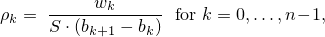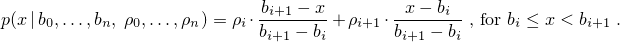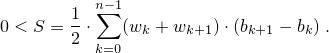# 26 Numerics library [numerics]

## 26.5 Random number generation [rand]

### 26.5.8 Random number distribution class templates [rand.dist]

#### 26.5.8.6.1 Class template discrete_distribution[rand.dist.samp.discrete]

A discrete_distribution random number distribution produces random integers i, 0 ≤ i < n, distributed according to the discrete probability function P(i | p0,…,pn-1) = pi .

Unless specified otherwise, the distribution parameters are calculated as: pk = wk / S for k = 0, …, n-1 , in which the values wk, commonly known as the weights, shall be non-negative, non-NaN, and non-infinity. Moreover, the following relation shall hold: 0 < S = w0 + ⋯ + wn-1 .

template<class IntType = int>
class discrete_distribution{
public:
// types
typedef IntType result_type;
typedef unspecified param_type;

// constructor and reset functions
discrete_distribution();
template<class InputIterator>
discrete_distribution(InputIterator firstW, InputIterator lastW);
discrete_distribution(initializer_list<double> wl);
template<class UnaryOperation>
discrete_distribution(size_t nw, double xmin, double xmax, UnaryOperation fw);
explicit discrete_distribution(const param_type& parm);
void reset();

// generating functions
template<class URNG>
result_type operator()(URNG& g);
template<class URNG>
result_type operator()(URNG& g, const param_type& parm);

// property functions
vector<double> probabilities() const;
param_type param() const;
void param(const param_type& parm);
result_type min() const;
result_type max() const;
};


 discrete_distribution(); 

Effects: Constructs a discrete_distribution object with n = 1 and p0 = 1 . [ Note: Such an object will always deliver the value 0.  — end note ]

 template<class InputIterator> discrete_distribution(InputIterator firstW, InputIterator lastW); 

Requires: InputIterator shall satisfy the requirements of an input iterator (Table [tab:iterator.input.requirements]) type. Moreover, iterator_traits<InputIterator>::value_type shall denote a type that is convertible to double. If firstW == lastW, let n = 1 and w0 = 1 . Otherwise, [firstW, lastW) shall form a sequence w of length n > 0.

Effects: Constructs a discrete_distribution object with probabilities given by the formula above.

 discrete_distribution(initializer_list<double> wl); 

Effects: Same as discrete_distribution(wl.begin(), wl.end()).

 template<class UnaryOperation> discrete_distribution(size_t nw, double xmin, double xmax, UnaryOperation fw); 

Requires: Each instance of type UnaryOperation shall be a function object ([function.objects]) whose return type shall be convertible to double. Moreover, double shall be convertible to the type of UnaryOperation's sole parameter. If nw = 0 , let n = 1 , otherwise let n = nw. The relation 0 < δ = (xmax - xmin) / n shall hold.

Effects: Constructs a discrete_distribution object with probabilities given by the formula above, using the following values: If nw = 0, let w0 = 1 . Otherwise, let wk = fw(xmin + k · δ + δ / 2) for k = 0, …, n-1 .

Complexity: The number of invocations of fw shall not exceed n.

 vector<double> probabilities() const; 

Returns: A vector<double> whose size member returns n and whose operator[] member returns pk when invoked with argument k for k = 0, …, n-1 .

#### 26.5.8.6.2 Class template piecewise_constant_distribution[rand.dist.samp.pconst]

A piecewise_constant_distribution random number distribution produces random numbers x, b0x < bn , uniformly distributed over each subinterval [ bi, bi+1 ) according to the probability density function p(x | b0,…,bn, ρ0,…,ρn-1) = ρi , for bix < bi+1 .

The n+1 distribution parameters bi, also known as this distribution's interval boundaries, shall satisfy the relation bi < bi+1 for i = 0, …, n-1 . Unless specified otherwise, the remaining n distribution parameters are calculated as:in which the values wk, commonly known as the weights, shall be non-negative, non-NaN, and non-infinity. Moreover, the following relation shall hold: 0 < S = w0 + ⋯ + wn-1 .

template<class RealType = double>
class piecewise_constant_distribution{
public:
// types
typedef RealType result_type;
typedef unspecified param_type;

// constructor and reset functions
piecewise_constant_distribution();
template<class InputIteratorB, class InputIteratorW>
piecewise_constant_distribution(InputIteratorB firstB, InputIteratorB lastB,
InputIteratorW firstW);
template<class UnaryOperation>
piecewise_constant_distribution(initializer_list<RealType> bl, UnaryOperation fw);
template<class UnaryOperation>
piecewise_constant_distribution(size_t nw, RealType xmin, RealType xmax, UnaryOperation fw);
explicit piecewise_constant_distribution(const param_type& parm);
void reset();

// generating functions
template<class URNG>
result_type operator()(URNG& g);
template<class URNG>
result_type operator()(URNG& g, const param_type& parm);

// property functions
vector<result_type> intervals() const;
vector<result_type> densities() const;
param_type param() const;
void param(const param_type& parm);
result_type min() const;
result_type max() const;
};


 piecewise_constant_distribution(); 

Effects: Constructs a piecewise_constant_distribution object with n = 1 , ρ0 = 1 , b0 = 0 , and b1 = 1 .

 template<class InputIteratorB, class InputIteratorW> piecewise_constant_distribution(InputIteratorB firstB, InputIteratorB lastB, InputIteratorW firstW); 

Requires: InputIteratorB and InputIteratorW shall each satisfy the requirements of an input iterator (Table [tab:iterator.input.requirements]) type. Moreover, iterator_traits<InputIteratorB>::value_type and iterator_traits<InputIteratorW>::value_type shall each denote a type that is convertible to double. If firstB == lastB or ++firstB == lastB, let n = 1 , w0 = 1 , b0 = 0 , and b1 = 1 . Otherwise, [firstB, lastB) shall form a sequence b of length n+1, the length of the sequence w starting from firstW shall be at least n, and any wk for kn shall be ignored by the distribution.

Effects: Constructs a piecewise_constant_distribution object with parameters as specified above.

 template<class UnaryOperation> piecewise_constant_distribution(initializer_list<RealType> bl, UnaryOperation fw); 

Requires: Each instance of type UnaryOperation shall be a function object ([function.objects]) whose return type shall be convertible to double. Moreover, double shall be convertible to the type of UnaryOperation's sole parameter.

Effects: Constructs a piecewise_constant_distribution object with parameters taken or calculated from the following values: If bl.size() < 2, let n = 1, w0 = 1 , b0 = 0 , and b1 = 1 . Otherwise, let [bl.begin(), bl.end()) form a sequence b0, …, bn , and let wk = fw((bk+1 + bk) / 2) for k = 0, …, n-1 .

Complexity: The number of invocations of fw shall not exceed n.

 template<class UnaryOperation> piecewise_constant_distribution(size_t nw, RealType xmin, RealType xmax, UnaryOperation fw); 

Requires: Each instance of type UnaryOperation shall be a function object ([function.objects]) whose return type shall be convertible to double. Moreover, double shall be convertible to the type of UnaryOperation's sole parameter. If nw = 0 , let n = 1 , otherwise let n = nw. The relation 0 < δ = (xmax - xmin) / n shall hold.

Effects: Constructs a piecewise_constant_distribution object with parameters taken or calculated from the following values: Let bk = xmin + k · δ for k = 0, …, n , and wk = fw(bk + δ / 2) for k = 0, …, n-1 .

Complexity: The number of invocations of fw shall not exceed n.

 vector<result_type> intervals() const; 

Returns: A vector<result_type> whose size member returns n + 1 and whose operator[] member returns bk when invoked with argument k for k = 0, …, n .

 vector<result_type> densities() const; 

Returns: A vector<result_type> whose size member returns n and whose operator[] member returns ρk when invoked with argument k for k = 0, …, n-1 .

#### 26.5.8.6.3 Class template piecewise_linear_distribution[rand.dist.samp.plinear]

A piecewise_linear_distribution random number distribution produces random numbers x, b0x < bn , distributed over each subinterval [ bi, bi+1 ) according to the probability density functionThe n+1 distribution parameters bi, also known as this distribution's interval boundaries, shall satisfy the relation bi < bi+1 for i = 0, …, n-1 . Unless specified otherwise, the remaining n+1 distribution parameters are calculated as ρk = wk / S for k = 0, …, n , in which the values wk, commonly known as the weights at boundaries, shall be non-negative, non-NaN, and non-infinity. Moreover, the following relation shall hold:template<class RealType = double>
class piecewise_linear_distribution{
public:
// types
typedef RealType result_type;
typedef unspecified param_type;

// constructor and reset functions
piecewise_linear_distribution();
template<class InputIteratorB, class InputIteratorW>
piecewise_linear_distribution(InputIteratorB firstB, InputIteratorB lastB,
InputIteratorW firstW);
template<class UnaryOperation>
piecewise_linear_distribution(initializer_list<RealType> bl, UnaryOperation fw);
template<class UnaryOperation>
piecewise_linear_distribution(size_t nw, RealType xmin, RealType xmax, UnaryOperation fw);
explicit piecewise_linear_distribution(const param_type& parm);
void reset();

// generating functions
template<class URNG>
result_type operator()(URNG& g);
template<class URNG>
result_type operator()(URNG& g, const param_type& parm);

// property functions
vector<result_type> intervals() const;
vector<result_type> densities() const;
param_type param() const;
void param(const param_type& parm);
result_type min() const;
result_type max() const;
};


 piecewise_linear_distribution(); 

Effects: Constructs a piecewise_linear_distribution object with n = 1 , ρ0 = ρ1 = 1 , b0 = 0 , and b1 = 1 .

 template<class InputIteratorB, class InputIteratorW> piecewise_linear_distribution(InputIteratorB firstB, InputIteratorB lastB, InputIteratorW firstW); 

Requires: InputIteratorB and InputIteratorW shall each satisfy the requirements of an input iterator (Table [tab:iterator.input.requirements]) type. Moreover, iterator_traits<InputIteratorB>::value_type and iterator_traits<InputIteratorW>::value_type shall each denote a type that is convertible to double. If firstB == lastB or ++firstB == lastB, let n = 1 , ρ0 = ρ1 = 1 , b0 = 0 , and b1 = 1 . Otherwise, [firstB, lastB) shall form a sequence b of length n+1, the length of the sequence w starting from firstW shall be at least n+1, and any wk for kn+1 shall be ignored by the distribution.

Effects: Constructs a piecewise_linear_distribution object with parameters as specified above.

 template<class UnaryOperation> piecewise_linear_distribution(initializer_list<RealType> bl, UnaryOperation fw); 

Requires: Each instance of type UnaryOperation shall be a function object ([function.objects]) whose return type shall be convertible to double. Moreover, double shall be convertible to the type of UnaryOperation's sole parameter.

Effects: Constructs a piecewise_linear_distribution object with parameters taken or calculated from the following values: If bl.size() < 2, let n = 1, ρ0 = ρ1 = 1 , b0 = 0 , and b1 = 1 . Otherwise, let [bl.begin(), bl.end()) form a sequence b0, …, bn , and let wk = fw(bk) for k = 0, …, n .

Complexity: The number of invocations of fw shall not exceed n+1.

 template<class UnaryOperation> piecewise_linear_distribution(size_t nw, RealType xmin, RealType xmax, UnaryOperation fw); 

Requires: Each instance of type UnaryOperation shall be a function object ([function.objects]) whose return type shall be convertible to double. Moreover, double shall be convertible to the type of UnaryOperation's sole parameter. If nw = 0 , let n = 1 , otherwise let n = nw. The relation 0 < δ = (xmax - xmin) / n shall hold.

Effects: Constructs a piecewise_linear_distribution object with parameters taken or calculated from the following values: Let bk = xmin + k · δ for k = 0, …, n , and wk = fw(bk) for k = 0, …, n .

Complexity: The number of invocations of fw shall not exceed n+1.

 vector<result_type> intervals() const; 

Returns: A vector<result_type> whose size member returns n + 1 and whose operator[] member returns bk when invoked with argument k for k = 0, …, n .

 vector<result_type> densities() const; 

Returns: A vector<result_type> whose size member returns n and whose operator[] member returns ρk when invoked with argument k for k = 0, …, n .